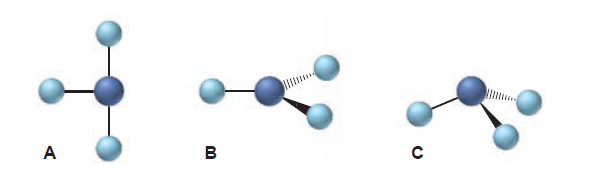×
Get Full Access to Principles Of General Chemistry - 2 Edition - Chapter 10 - Problem 81p
Get Full Access to Principles Of General Chemistry - 2 Edition - Chapter 10 - Problem 81p

×

# Consider the following molecular shapes: (a) Match eachISBN: 9780073511085 71

## Solution for problem 81P Chapter 10

Principles of General Chemistry | 2nd Edition

• Textbook Solutions
• 2901 Step-by-step solutions solved by professors and subject experts
• Get 24/7 help from StudySoup virtual teaching assistantsPrinciples of General Chemistry | 2nd Edition

4 5 1 245 Reviews
20
2
Problem 81P

Consider the following molecular shapes:(a) Match each shape with one of the following species: XeF3+, SbBr3, GaCl3.

(b) Which, if any, is polar?

(c) Which has the most valence electrons around the central atom?

Step-by-Step Solution:

Solution 81P:

Step 1: (a)

Here, we are going to match the shape of the species.

For determining the shape, we have to use  VSEPR theory.

The valence-shell electron-pair repulsion (VSEPR) theory is based on the electron groups around the central atom.

An electron group can be an electron pair, a lone pair, a single unpaired electron, a double bond or a triple bond on the center atom. VSEPR theory can help to predict the shape of a molecule.

According to VSEPR theory,

1. The geometry of a molecule is determined by the number of electron groups on the central atom.
2. The number of electron groups is determined from the Lewis structure of the molecule.
3. The geometry of the electron groups is determined by their repulsions

Lone pair–lone pair >  lone pair–bonding pair >  bonding pair–bonding pair

Lewis structure of  XeF3+:

Valence electrons of Xe is 8 (Group VIIIA)

Valence electrons of F is 7 (Group VIIA)

Total valence electrons of XeF3+ is (37 +8 -1 =28)Here, each Xe atom is surrounded by five electron groups around it. Therefore, its electron-group arrangement is trigonal bipyramidal. Thus, the molecular shape of XeF3+ is T-shape due to presence of lone pair of electrons

Therefore, the ideal angle for F-Xe-F is <90o.

Thus, XeF3+  matches with the diagram A.

Step 2 of 5

Step 3 of 5

##### ISBN: 9780073511085

Unlock Textbook Solution